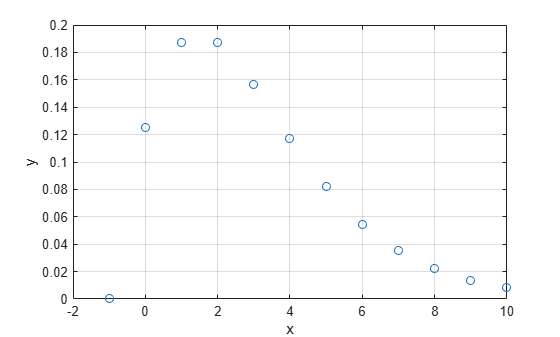# nbinpdf

Negative binomial probability density function

## Syntax

```Y = nbinpdf(X,R,P) ```

## Description

`Y = nbinpdf(X,R,P)` returns the negative binomial pdf at each of the values in `X` using the corresponding number of successes, `R` and probability of success in a single trial, `P`. `X`, `R`, and `P` can be vectors, matrices, or multidimensional arrays that all have the same size, which is also the size of `Y`. A scalar input for `X`, `R`, or `P` is expanded to a constant array with the same dimensions as the other inputs. Note that the density function is zero unless the values in `X` are integers.

The negative binomial pdf is

`$y=f\left(x|r,p\right)=\left(\begin{array}{c}r+x-1\\ x\end{array}\right){p}^{r}{q}^{x}{I}_{\left(0,1,...\right)}\left(x\right)$`

The simplest motivation for the negative binomial is the case of successive random trials, each having a constant probability `P` of success. The number of extra trials you must perform in order to observe a given number `R` of successes has a negative binomial distribution. However, consistent with a more general interpretation of the negative binomial, `nbinpdf` allows `R` to be any positive value, including nonintegers. When `R` is noninteger, the binomial coefficient in the definition of the pdf is replaced by the equivalent expression

`$\frac{\Gamma \left(r+x\right)}{\Gamma \left(r\right)\Gamma \left(x+1\right)}$`

## Examples

collapse all

Compute the pdf of a negative binomial distribution with parameters `R = 3` and `p = 0.5`.

```x = (0:10); y = nbinpdf(x,3,0.5);```

Plot the pdf.

```figure; plot(x,y,'+') xlim([-0.5,10.5])```## Version History

Introduced before R2006a